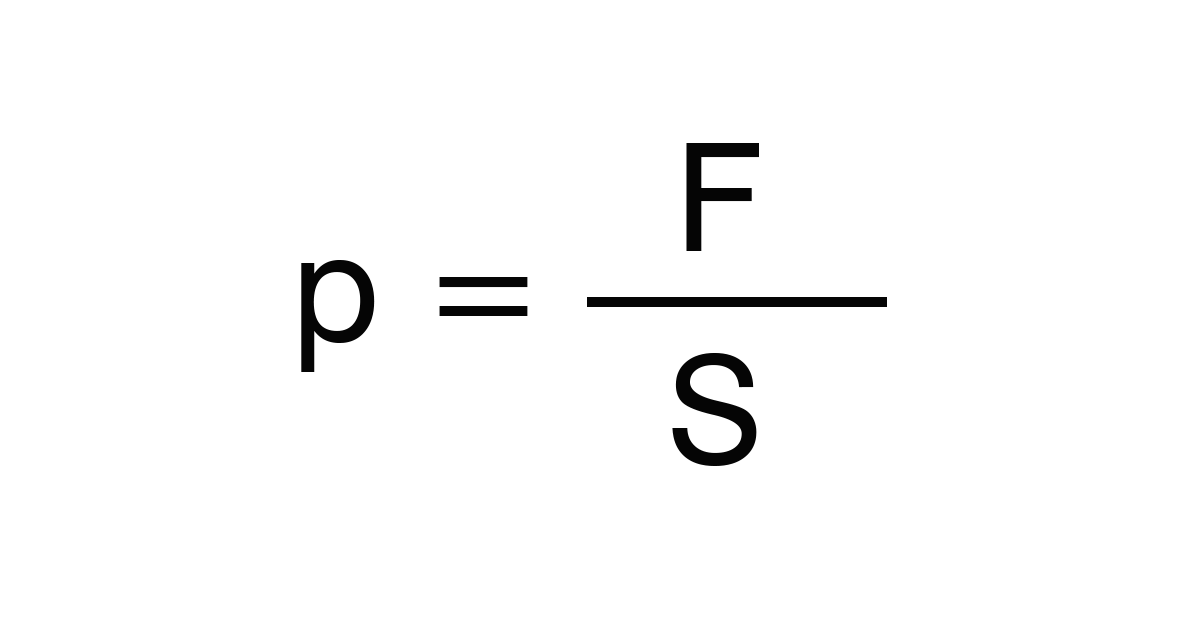﻿ Pressure force: - on line calculation, formula - FORMIAX

# PressureF = Pressure force

S = surface

WHAT IS IT ?
Pressure is the force applied perpendicular to the surface of an object per unit area.

CALCULATION:

Enter pressure force

Enter surface

Round to number of decimals places

YOU MIGHT BE INTERESTED: